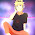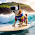## Word Break I

Given a string s and a dictionary of words dict, determine if s can be segmented into a space-separated sequence of one or more dictionary words.
For example, given
s = "leetcode",
dict = ["leet", "code"].
Return true because "leetcode" can be segmented as "leet code".

### Analysis:

First thought is DFS (depth first search), searching every dict elements, if matched go to next position of s, until find the last match. Consider a very long s has a bunch of "a" and other letters, and the dict has "a", the searching will be pretty slow then.

Note that the problem asked for "if or not", which means we don't need to know which dicts compose string s, but only a "true/false" result.

Instead of search each element in the dict, search every position of the string is another way:
Say mp[i][j]=true if S[i to j] can be found in dict, mp[i][j]=false otherwise.  In this way, we now have a matrix (actually upper triangle of the matrix) which shows the initial matching of string S.

Next step, the question becomes:  find the value of mp[s.size()-1]. How to determine mp[i][j]?
We can see that, for all the mp[i][j]=false, if there exists a k (i<=k<j), mp[i][k]==true and mp[k+1][j]==true, then mp[i][j]=true. This means, if s[i to k] can be found in the dict, and, s[k+1 to j] can also be found in the dict, then s[i to j] is a valid match. In the code, just set mp[i][j]=mp[i][k]&&mp[k+1][j] will work well.

The complexity of this problem is O(n^3), where n is the length of the original string. The code below has been tested and passed all the test cases in the OJ.

### Code:

class Solution {
public:
bool wordBreak(string s, unordered_set<string> &dict) {
// IMPORTANT: Please reset any member data you declared, as
// the same Solution instance will be reused for each test case.
if (s.empty()){return false;}
vector<vector<bool> > mp(s.size(),vector<bool>(s.size(),false));

for (int i=0;i<s.size();i++){
for (int j=i;j<s.size();j++){
if (dict.find(s.substr(i,j-i+1))!=dict.end()){
mp[i][j]=true;
}
}
}

for (int i=0;i<s.size();i++){
for (int j=i;j<s.size();j++){
for(int k=i;k<j;k++){
if (!mp[i][j]){
mp[i][j]=mp[i][k]&&mp[k+1][j];
}
}
}
}

return mp[s.size()-1];
}
};


1.first part is great. but in the second part, why not use dynamic programming, just as you did in your other posts? complexity reduced to O(n*n)

here is my solution
http://westpavilion.blogspot.com/2014/01/leetcode-word-break.html

2.Hello, I think the second part could be:
int i = 0;
for (int j=i;j<s.size();j++){
for(int k=i;k<j;k++){
if (!mp[i][j]){
mp[i][j]=mp[i][k]&&mp[k+1][j];
}
}
}

1.I agree with you. i=0 is all we need.

3.Hi, two dimension array is not needed in this problem. Take a look at my solution below.

public boolean wordBreak(String s, Set dict) {
if(s.isEmpty()) return true;
else if(dict.isEmpty()) return false;
boolean[] dp=new boolean[s.length()];
for(int i=0;i=0;j--)
{
String cur=s.substring(j,i+1);
if((j==0||dp[j-1])&&dict.contains(cur)) {dp[i]=true;break;}
}
}
return dp[s.length()-1];

}

4.Can you please post an Trie solution, I am really struggling with the test cases and over use of search method in the trie.